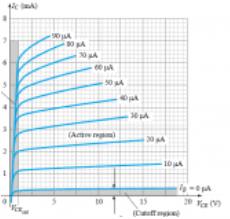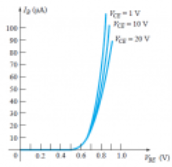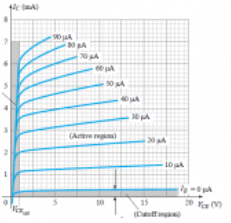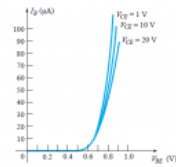Courses

Test: BJT Configuration

10 Questions MCQ Test Analog Circuits | Test: BJT Configuration

Description
Attempt Test: BJT Configuration | 10 questions in 10 minutes | Mock test for Electronics and Communication Engineering (ECE) preparation | Free important questions MCQ to study Analog Circuits for Electronics and Communication Engineering (ECE) Exam | Download free PDF with solutions
QUESTION: 1

For a BJT, for common base configuration the input characteristics is represented by a plot between which of the following parameters?

Solution:

For a BJT, the input characteristics is given by a plot between VBE and IB.

QUESTION: 2

For a BJT, for common base configuration the output characteristics is represented by a plot between which of the following parameters?

Solution:

For a BJT, the output characteristics is given by a plot between VCE and IC.

QUESTION: 3

In a BJT, if the collector-base junction is reverse-biased and the base-emitter junction is forward-biased, which region is the BJT operating in?

Solution:

If the collector-base junction is reverse-biased and the base-emitter junction is forward-biased,then the BJT functions in the active region of the output characteristics.

QUESTION: 4

In a BJT, if the collector-base junction is forward-biased and the base-emitter junction is forward-biased, which region is the BJT operating in?

Solution:

If the collector-base junction and the base-emitter junction are both forward-biased,then the BJT functions in the saturation region of the output characteristics.

QUESTION: 5

In a BJT, if the collector-base junction and the base-emitter junction are both reverse-biased, which region is the BJT operating in?

Solution:

If the collector-base junction and the base-emitter junction are both reverse-biased,then the BJT functions in the cutoff region of the output characteristics.

QUESTION: 6

From the given characteristics, what is the value of IC at IB=30 uA and VCE=10 V?Solution:

At the intersection of IB=30 uA and VCE=10 V, IC=3.4 mA.

QUESTION: 7

From the given characteristics, what is the value of IC at VBE=0.7 V and VCE=15 V?Solution:

From the characteristics, the value of IB at VBE=0.7V is 20uA. Now, from IB=20 uA, we get IC = 2.5 mA.

QUESTION: 8

Which of the following correctly determines the relation between α and β?

Solution:

α and β are related as β=α/(1-α).

QUESTION: 9

The value of IC is precisely zero when the value of IE is zero.

Solution:

When the value of IE is zero, then the value of IC is equal to ICBO which is in the order of microamperes but not zero.

QUESTION: 10

For common emitter configuration, which of the following is the correct relation?

Solution:

All the relations hold true i.e. IC = βIB and IC = αIE. As α<1, hence IC < IEUse Code STAYHOME200 and get INR 200 additional OFF Use Coupon Code

Track your progress, build streaks, highlight & save important lessons and more!

Similar ContentRelated tests